# Conductor of a character

An integer associated to the character of a representation of the Galois group of a finite extension of a local field. Letbe a field that is complete with respect to a discrete valuation, with residue class fieldof characteristic. Let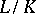be a Galois extension of degreewith Galois groupand suppose that the residue class field extension is separable. Ifis the character of some finite-dimensional complex representation of, its conductoris defined by the formula: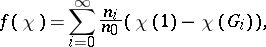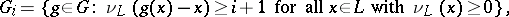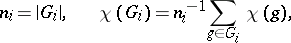whereis the corresponding valuation of. Ifdoes not divide, then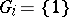for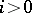and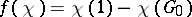. Ifis the character of a rational representation, then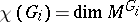. The conductor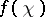is a non-negative integer.